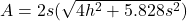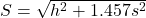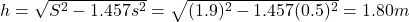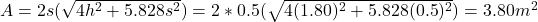## The roof of a gazebo is a regular octagonal pyramid. If the base of the pyramid has sides of 0.5 meters and the slant height of the roof is

Question

The roof of a gazebo is a regular octagonal pyramid. If the base of the pyramid has sides of 0.5 meters and the slant height of the roof is 1.9 meters, find the area of the roof. (roof is lateral surface area)

in progress 0
2 months 2021-08-22T11:30:11+00:00 1 Answers 1 views 0

The area of the roof is 3.80 m².

Step-by-step explanation:

The lateral surface area of an octagonal pyramid is given by:(1)

Where:

s: is the length of the base edge = 0.5 m

h: is the height

We can find the height from the slant height (S = 1.9 m):(2)

By solving equation (2) for “h” we have:Now, we can calculate the area of the roof (equation 1):Therefore, the area of the roof is 3.80 m².

I hope it helps you!